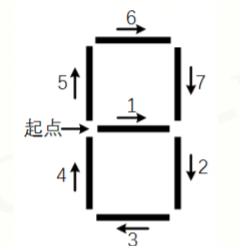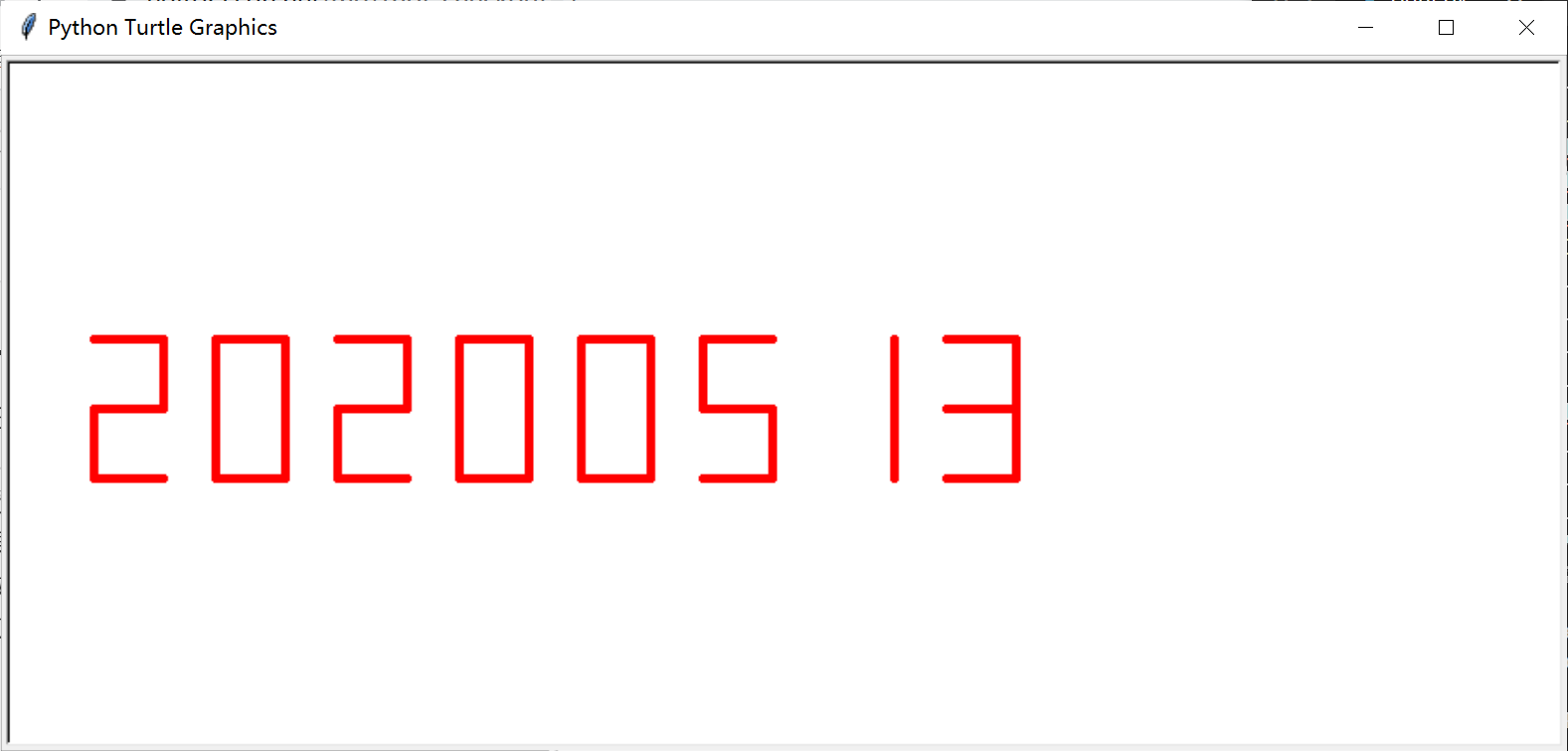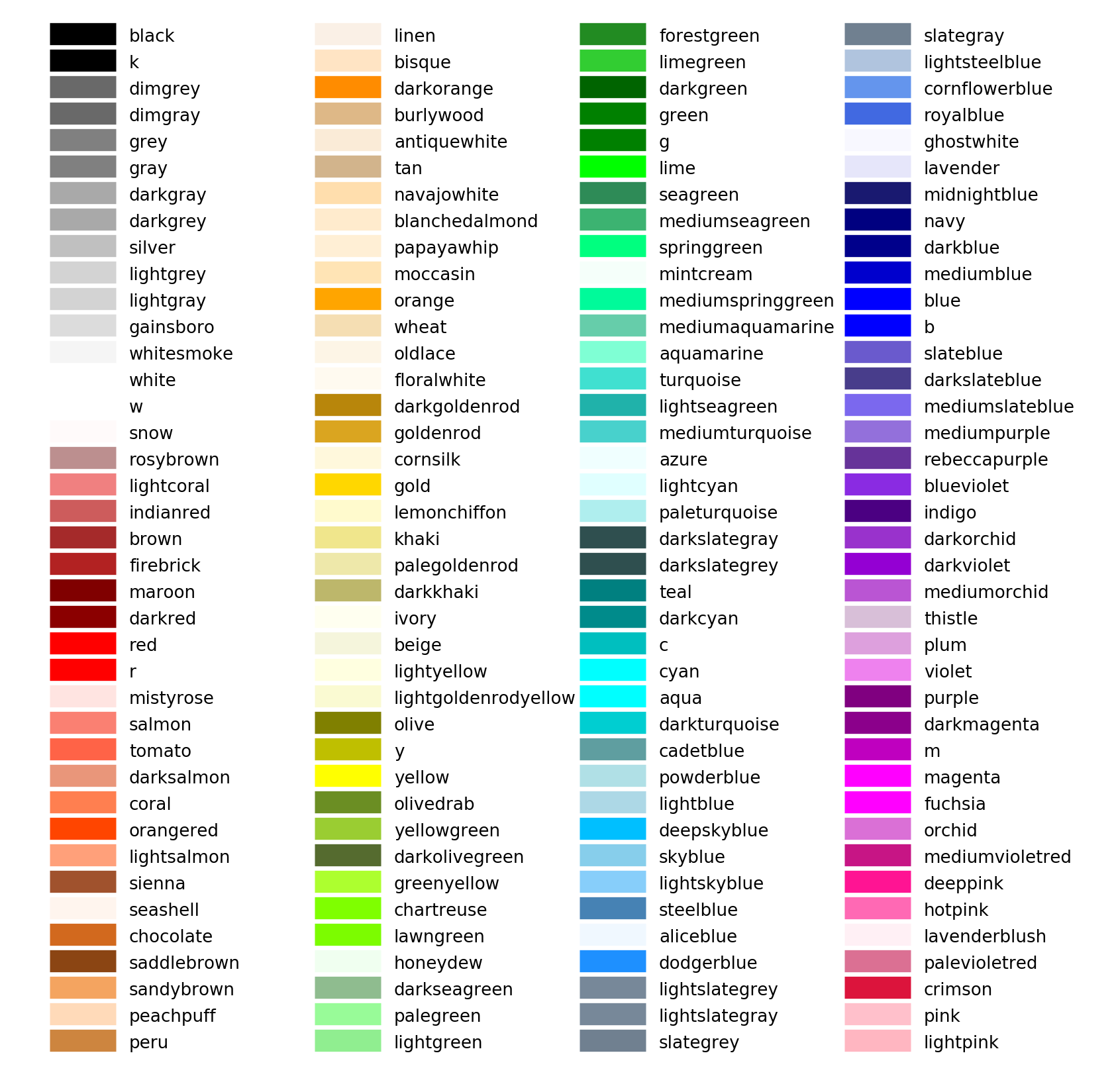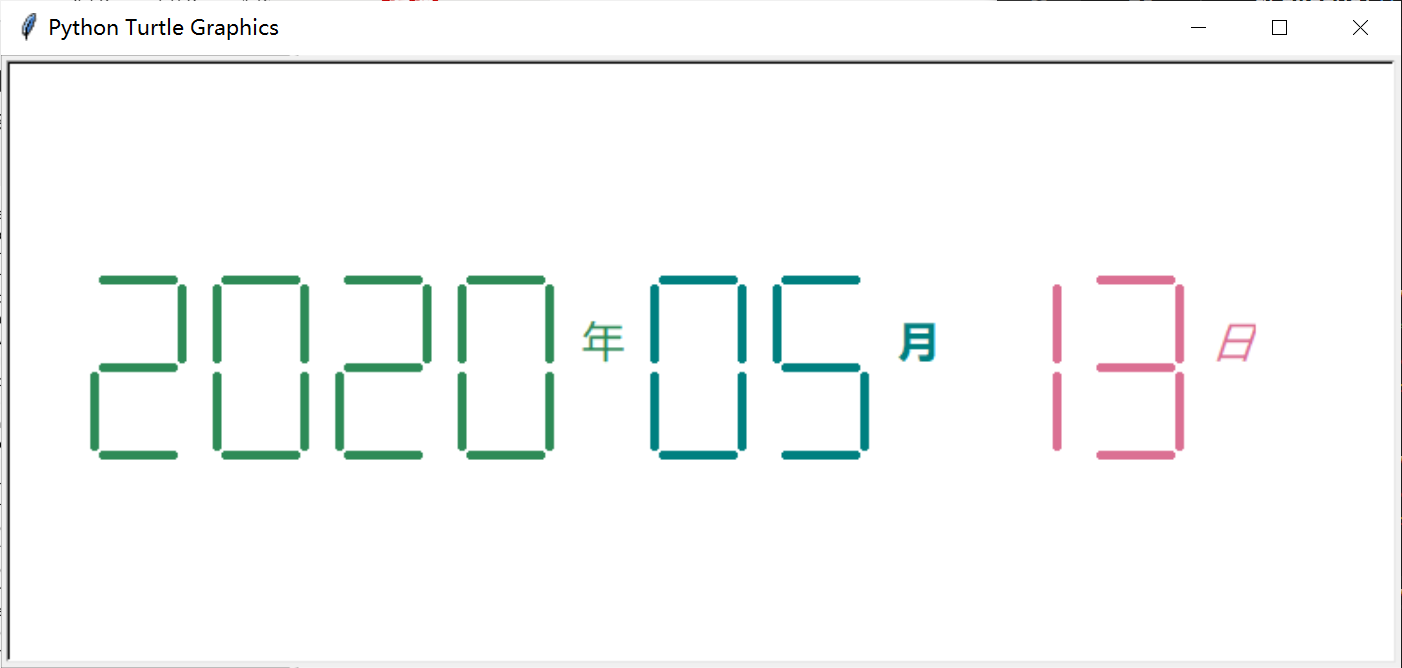# python七段数码管的绘制 获取系统时间

2020-06-29 05:04 169 查看

## Lily学python## 第一个无聊数码管

****

```import turtle as t, datetime as d #对于懒癌患者，不缩写是不可能的
def drawLine(draw):   #绘制单段数码管
t.pd() if draw else t.pu() #紧凑分支结构 #pendown、penup也不配拥有全名
t.fd(40)
t.right(90)
def drawDigit(di): #根据数字绘制七段数码管，di接收数字，永远对应着七段绘制
drawLine(True) if di in [2,3,4,5,6,8,9] else drawLine(False)
#每一笔画不画，都要考虑一下
drawLine(True) if di in [0,1,3,4,5,6,7,8,9] else drawLine(False)
drawLine(True) if di in [0,2,3,5,6,8,9] else drawLine(False)
drawLine(True) if di in [0,2,6,8] else  drawLine(False)
t.left(90) #画完第四段，调整为向北的方向
drawLine(True) if di in [0,4,5,6,8,9] else drawLine(False)
drawLine(True) if di in [0,2,3,5,6,7,8,9] else drawLine(False)
drawLine(True) if di in [0,1,2,3,4,7,8,9] else drawLine(False)
t.left(180)#为后续数字确定方向
t.pu()
t.fd(30)#为后续数字确定位置
def drawDate(date):  #获得要输出的数字
for i in date:
drawDigit(eval(i))  #注意: 通过eval()函数将数字变为整数
def main():
t.setup(900, 400, 50, 50)#设置画布大小、位置
t.pu()
t.fd(-400)
t.pencolor('red')#来个最容易写的红色
t.pensize(5)
drawDate(d.datetime.now().strftime('%Y%m%d'))
#'%Y'输出2020这样的四位“年号”，hey不要误解，我历史没白学
#'%m%d'顾名思义啦，month、day，极其中规中矩，可以试试把大小写换掉哦

t.hideturtle()#快快快，把你的小尾巴藏起来
main()
t.done()#建议要有这一行哦，否则啊，画完就逃走```

**

## 升阶例子 time库获得系统时间，增加“年月日”标记，年月日颜色不同

**po代码：

```import turtle as t, datetime as d,time

def drawGap(): #绘制数码管间隔
t.penup()
t.fd(5)
def drawLine(draw):   #绘制单段数码管
drawGap()
t.pd() if draw else t.pu()
t.fd(40)
drawGap()
t.right(90)
def drawDigit(di): #根据数字绘制七段数码管
drawLine(True) if di in [2,3,4,5,6,8,9] else drawLine(False)
drawLine(True) if di in [0,1,3,4,5,6,7,8,9] else drawLine(False)
drawLine(True) if di in [0,2,3,5,6,8,9] else drawLine(False)
drawLine(True) if di in [0,2,6,8] else drawLine(False)
t.left(90)
drawLine(True) if di in [0,4,5,6,8,9] else drawLine(False)
drawLine(True) if di in [0,2,3,5,6,7,8,9] else drawLine(False)
drawLine(True) if di in [0,1,2,3,4,7,8,9] else drawLine(False)
t.left(180)
t.pu()
t.fd(20)
def drawDate(date):
t.pencolor("seagreen")#啊我就是喜欢不一样的颜色名
for i in date:
if i == '-':#自己给出的+-=，方便处理
t.write('年',font=("微软雅黑", 18, "normal"))#输出汉字字体，字号，字形（斜体、加粗……）
#没错我就是要用字体的中文名，多么感动，如果你电脑里有“方正孙拥声简体”之类的，无妨一试
t.pencolor("teal")
t.fd(40)
elif i == '=':
t.write('月',font=("微软雅黑", 18, "bold"))#加粗
t.pencolor("palevioletred")
t.fd(40)
elif i == '+':
t.write('日',font=("微软雅黑", 18, "italic"))#斜体
else:
drawDigit(eval(i))
def main():
t.setup(800, 350, 200, 200)
t.pu()
t.fd(-350)
t.pensize(5)
drawDate(d.datetime.now().strftime('%Y-%m=%d+'))
#strftime表示按我们所需要的的形式
# %y两位数的年份表示(00-99)
# %Y四位数的年份表示(000-9999)
# %m月份(01-12)
# %d月内中的一天(0-31)
t.hideturtle()
main()
t.done()```